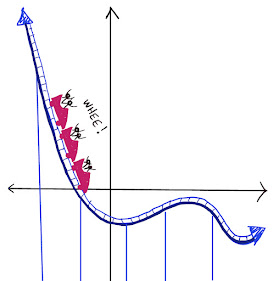## Tuesday, March 24, 2020

### The Funny Calculator

Just like a “normal” calculator,

When you type 1 x 10, it says 10.
When you type 1 + 10, it says 11.

But…

When you type 1 + 1, it says 10.
When you type 10 + 10, it says 100.

--------------------------------------------------------------------------------------------

Using this information alone:

How would you count from one to four?

What would the calculator say if you typed in 100 10 ?

Extending the pattern:

Can you extend the pattern to count up to twenty?

What would the calculator say if you typed in 0.1 + 0.1?

What would the calculator say if you typed in 0.1 x 10 ?

What would the calculator say if you typed in 111 + 111 ?

What would the calculator say if you typed in 111 x 10 ?

### For the solution, click "Read More" below.Solutions:

In general, this number system is a base 2 system. The puzzle is more interesting if you’ve never studied the base 2 number system and have to work the system out logically from the statements above. Even if you have studied the base 2 number system, this may give you a new way to think about it.

Using this information alone:

• How would you count from one to four?

1, 10, 11, 100

10 equals two because 1 + 1 = 10

11 equals three because 10 + 1 = 11

100 equals four because 10 + 10 = 100

• What would the calculator say if you typed in 100 10 ?

Four divided by two equals two, so it would say 100 10 = 10, just like a “normal” calculator.

Extending the pattern:

• Can you extend the pattern to count up to twenty?

1, 10, 11, 100, 101, 110, 111, 1000, 1001, 1010, 1011, 1100, 1101, 1110, 1111, 10000, 10001, 10010, 10011, 10100

• What would the calculator say if you typed in 0.1 + 0.1?

Doubling a number scoots the “decimal” point over one, so

0.1 + 0.1 = 1 and 0.1 has the value of one half.

What would the calculator say if you typed in 0.1 x 10 ?

Multiplying by “10” is doubling in this number system, which means scooting the “decimal” point over once.  So:

0.1 + 0.1   =    0.1 x 10    =     1

• What would the calculator say if you typed in 111 + 111 ?

Doubling a number scoots the decimal point over one, so 111 + 111 = 1110.

• What would the calculator say if you typed in 111 x 10 ?

Multiplying by “10” is doubling in this number system, which means scooting the “decimal” point over once.  So:

111 + 111   =    111 x 10    =     1110